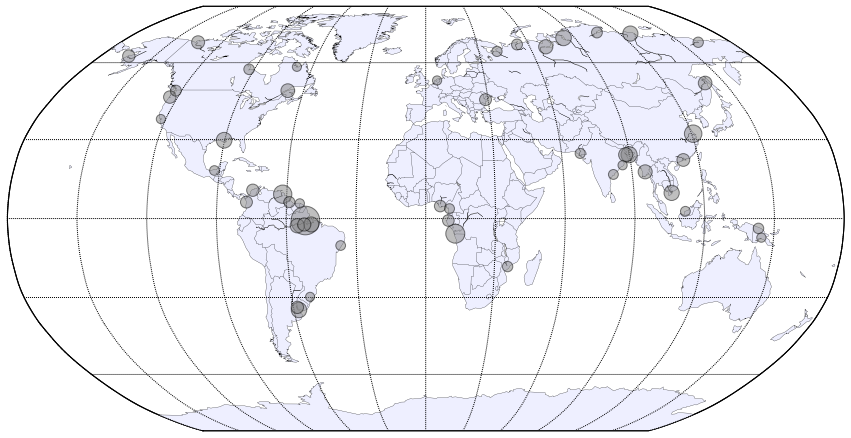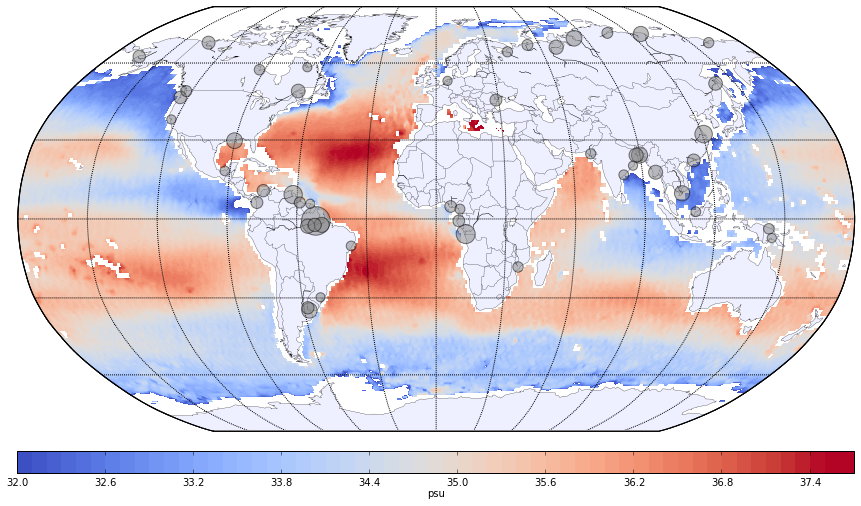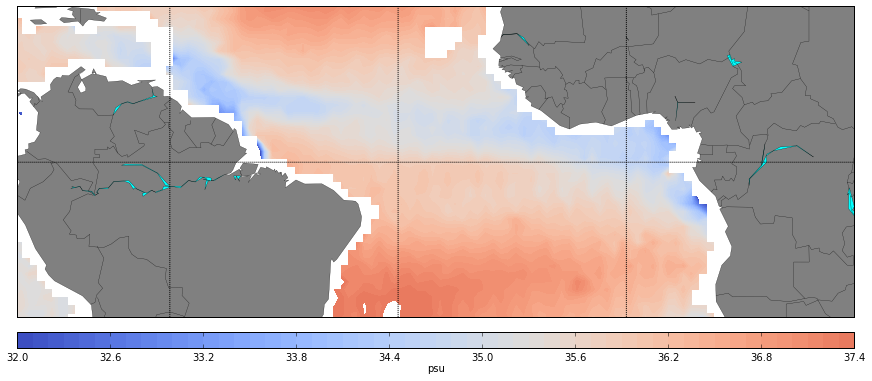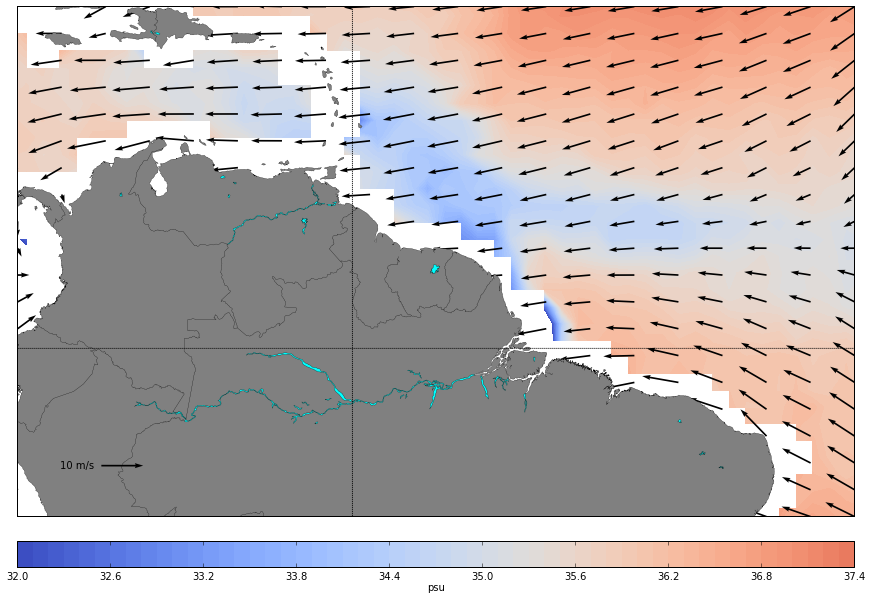# Amazon river discharge¶

I want to show a small example of the work flow that is more or less typical during research process. Often you see some interesting feature in your data and want to investigate it in more detail. If you not lucky enough to work with the model data, this would require dealing with multiple data sources, and possibly multiple file formats. Having all data sets in one place in consistent format becomes very handy for this type of applications.

I begin with the visualization of river discharge data, that I did for one of my projects. It is performed in Tableau, and use Dai and Trenberth Global River Flow and Continental Discharge Dataset. This data set is also available on Marinexplore.

In :
from IPython.display import HTML
HTML('<iframe src=http://public.tableausoftware.com/views/rivers_0/top925rivers?:embed=y&:display_count=no width=800 height=700></iframe>')

Out:

This visualization is interactive, so if you choose several rivers on the map it will show a bar chart with distribution of river discharges.

I also want to show some simple statistics about my data, so I create another visualization with only 20 biggest rivers:

In :
HTML('<iframe src=http://public.tableausoftware.com/views/rivers_0/top20rivers width=800 height=700></iframe>')

Out:

Look at Amazon. I knew that it's a biggest river in the world, but I never though it's domination is so overwhelming. This huge river discharge should be visible somehow in salinity field of the ocean. In order to test this I decide to look at satellite salinity data.

But first we would need to plot our river discharge data in pyhton. Text file with anual mean river discharge values from Dai and Trenberth have been converted in .csv format with vertical bar as separator and is available here. We are going to open it with pandas, and plot with basemap.

In :
%pylab inline

Welcome to pylab, a matplotlib-based Python environment [backend: module://IPython.kernel.zmq.pylab.backend_inline].

In :
import pandas as pd

In :
df = pd.read_csv('rivers2.csv', sep='|', na_values=[' Null', '  Null'])


Here is our data in pandas DataFrame:

In :
df.head()

Out:
No m2s_ra lonm latm area(km2) Vol nyr yrb yre elev(m) CT CN River_Name OCN Station_Name
0 1 12462 -51.75 -0.75 4618750 5389.537 79.0 1928 2006 37 BR SA Amazon ATL Obidos, Bra
1 2 10291 12.75 -5.75 3475000 1270.203 97.1 1903 2000 270 CD AF Congo ATL Kinshasa, C
2 3 11474 -61.75 9.25 836000 980.088 75.8 1923 1999 -9999 VE SA Orinoco ATL Pte Angostu
3 4 10374 120.75 32.25 1705383 905.141 99.6 1900 2000 19 CN AS Changjiang PAC Datong, Chi
4 5 10245 89.75 24.25 554542 670.943 44.3 1956 2000 19 BD AS Brahmaputra IND Bahadurabad

We need only several values to work with:

In :
df_data = df[[' lonm', 'latm',  'Vol', 'm2s_ra']]


We don't need missing values:

In :
df_data = df_data.dropna()


And convert values to floats:

In :
df_data['Vol'] = df_data['Vol'].astype('float32')
df_data['lonm'] = df_data[' lonm'].astype('float32')
df_data['latm'] = df_data['latm'].astype('float32')
df_data['m2s_ra'] = df_data['m2s_ra'].astype('float32')


Now plot the map:

In :
from mpl_toolkits.basemap import Basemap
import matplotlib.cm as cm

In :
m = Basemap(projection='robin',lon_0=0,resolution='c')
x, y = m(df_data['lonm'][0:50], df_data['latm'][0:50])

In :
figure(figsize=(15,15))
m.drawcoastlines(linewidth=0.25)
m.drawcountries(linewidth=0.25)

m.fillcontinents(color='#eeefff',lake_color='white')
m.drawmapboundary(fill_color='white')
m.drawmeridians(np.arange(0,360,30))
m.drawparallels(np.arange(-90,90,30))
m.scatter(x,y,s=np.sqrt(df_data['Vol'][0:50]*(df_data['m2s_ra'][0:50]/10000))*10,c='gray',marker='o',zorder=4,alpha=0.5)

Out:
<matplotlib.collections.PathCollection at 0xb86ef4c>This is discharge of first 50 rivers from Dai and Trenberth data set. Let's add some salinity.

In order to do so I first generated subset of the AQUARIUS sea surface salinity data. The data set is very short, so I took only one year (2012). I also need only annual mean, and had to generate it by myself with use of cdo. At this point I encountered some probelms. The output is in netCDF4, that is still not very abundant, and soft for handling netCDF files in standard linux distributions (like cdo, or scipy.io.netcdf) is still compiled without netCDF4 support. This is a bit annoying, and it would be nice to be able to choose between netCDF3 and 4.

The file with annual mean sea surface salinity for 2012 is available from here and it's nerCDF3. I use Nio to open it, but it should be very similar to scipy.io.netcdf.

In :
import Nio

In :
ff = Nio.open_file('AQUARIUS.L3.7DAY_tm.nc')
sal = ff.variables['sea_water.salinity'][:]
lat = ff.variables['lat'][:]
lon = ff.variables['lon'][:]

In :
lon, lat = np.meshgrid(lon, lat)

In :
import matplotlib.cm as cm

In :
m2 = Basemap(projection='robin',lon_0=0,resolution='c')
x2, y2 = m2(lon,lat)

In :
figure(figsize=(15,15))
m2.drawcoastlines(linewidth=0.25)
m2.drawcountries(linewidth=0.25)

m2.fillcontinents(color='#eeefff',lake_color='white')
m2.drawmapboundary(fill_color='white')
m2.drawmeridians(np.arange(0,360,30))
m2.drawparallels(np.arange(-90,90,30))
cs = m.contourf(x2,y2,sal[0,0,:,:], levels=np.arange(32,37.7,0.1), cmap=cm.coolwarm,  vmin=32, vmax=37.5 )
m2.scatter(x,y,s=np.sqrt(df_data['Vol'][0:50]*(df_data['m2s_ra'][0:50]/10000))*10,c='gray',marker='o',zorder=4, cmap=cm.Paired,alpha=0.5)
cbar.set_label('psu')One can see that there is some reduces salinity, however it is located not to the east of the Amazon river mouth, but more to the north of it. Let's have a closer look:

In :
m3 = Basemap(projection='merc',llcrnrlat=-20,urcrnrlat=20,\
llcrnrlon=-80,urcrnrlon=30,lat_ts=20,resolution='c')
x3, y3 = m3(lon,lat)

In :
figure(figsize=(15,15))
m3.drawcoastlines(linewidth=0.25)
m3.drawcountries(linewidth=0.25)

m3.fillcontinents(color='gray',lake_color='aqua')
m3.drawmapboundary(fill_color='white')
m3.drawmeridians(np.arange(0,360,30))
m3.drawparallels(np.arange(-90,90,30))
cs = m3.contourf(x3,y3,sal[0,0,:,:], levels=np.arange(32,37.5,0.1), cmap=cm.coolwarm,  vmin=32, vmax=38.5 )
cbar.set_label('psu')The fresh water plume is obviously going to the north, and then at some point partly turn to the east. There is also some signature of big rivers from the western coast of the African continent. However we will concentrate on the Amazon river plume. What is causing the shape of it's surface distribution? Maybe winds?

In order to test this I create data set with NCDC sea winds for 2012, and calculate annual mean (available from here).

In :
fw = Nio.open_file('NOAA.NCDC.SEAWINDS.RT.DAILY_tm.nc', 'r')


In order to be able to plot vectors with Basemap we have to flip our data (lat should be monotonically increasing):

In :
latw = np.flipud(fw.variables['lat'][:])
lonw = fw.variables['lon'][:]
uw = np.flipud(fw.variables['wind.vector_u'][0,0,:,:])
vw = np.flipud(fw.variables['wind.vector_v'][0,0,:,:])

In :
m4 = Basemap(projection='merc',llcrnrlat=-10,urcrnrlat=20,\
llcrnrlon=-80,urcrnrlon=-30,lat_ts=20,resolution='i')
x4, y4 = m4(lon,lat)

In :
uwproj,vwproj,xxw,yyw = \

In :
figure(figsize=(15,15))
m4.drawcoastlines(linewidth=0.25)
m4.drawcountries(linewidth=0.25)

m4.fillcontinents(color='gray',lake_color='aqua')
m4.drawmapboundary(fill_color='white')
m4.drawmeridians(np.arange(0,360,30))
m4.drawparallels(np.arange(-90,90,30))
cs = m4.contourf(x4,y4,sal[0,0,:,:], levels=np.arange(32,37.5,0.1), cmap=cm.coolwarm,  vmin=32, vmax=38.5 )
Q = m4.quiver(xxw,yyw,uwproj,vwproj,scale=200, width=0.002)
qk = plt.quiverkey(Q, 0.1, 0.1, 10, '10 m/s', labelpos='W')
#colorbar(orientation = 'horizontal')
cbar.set_label('psu')The mean wind is classical, like from the text books, and can't lead to such distribution. This was strange idea from the beginning, but sometimes it worth to test even strange ideas :)

So, what about ocean currents, how do they look like in this area?

Data set that we will use is here and time mean for 2012 is located here.

In :
fc = Nio.open_file('NOAA.OSCAR.CURRENTS_tm.nc', 'r')

In :
lats = np.flipud(fc.variables['lat'][:])
lons = fc.variables['lon'][:]
u = np.flipud(fc.variables['sea_water.current.vector_u'][0,0,:,:])
v = np.flipud(fc.variables['sea_water.current.vector_v'][0,0,:,:])

In :
lonuv, latuv = np.meshgrid(lons,lats)

In :
m5 = Basemap(projection='merc',llcrnrlat=-10,urcrnrlat=20,\
llcrnrlon=-80,urcrnrlon=-30,lat_ts=20,resolution='i')
x5, y5 = m5(lon,lat)

In :
uproj,vproj,xx,yy = \

In :
figure(figsize=(15,15))
m5.drawcoastlines(linewidth=0.25)
m5.drawcountries(linewidth=0.25)

m5.fillcontinents(color='gray',lake_color='aqua')
m5.drawmapboundary(fill_color='white')
m5.drawmeridians(np.arange(0,360,30))
m5.drawparallels(np.arange(-90,90,30))
cs = m5.contourf(x5,y5,sal[0,0,:,:], levels=np.arange(32,37.5,0.1), cmap=cm.coolwarm,  vmin=32, vmax=38.5 )
Q = m5.quiver(xx,yy,uproj,vproj,scale=10, width=0.0012)
qk = plt.quiverkey(Q, 0.1, 0.1, 0.1, '0.1 m/s', labelpos='W')
#colorbar(orientation = 'horizontal')